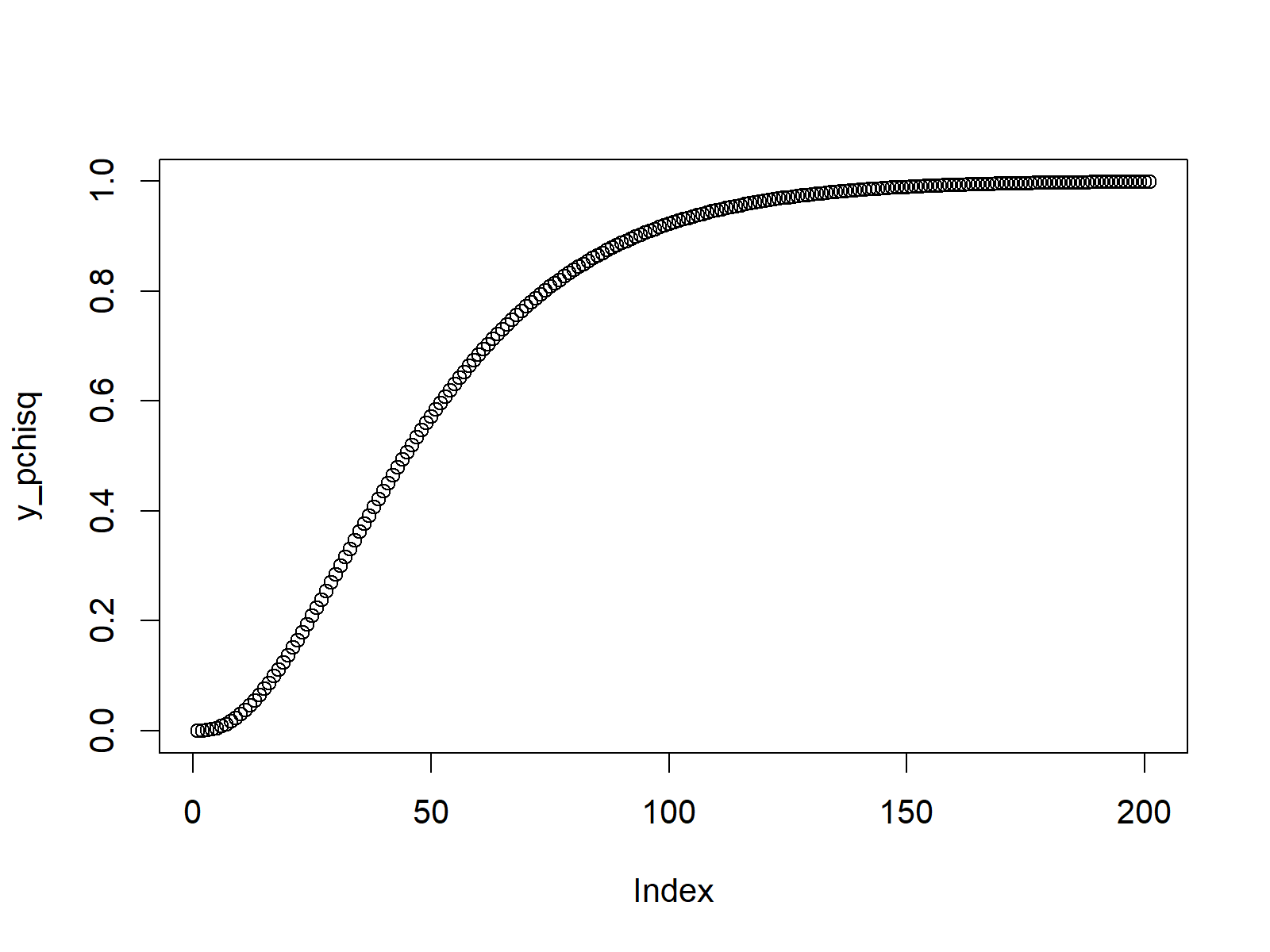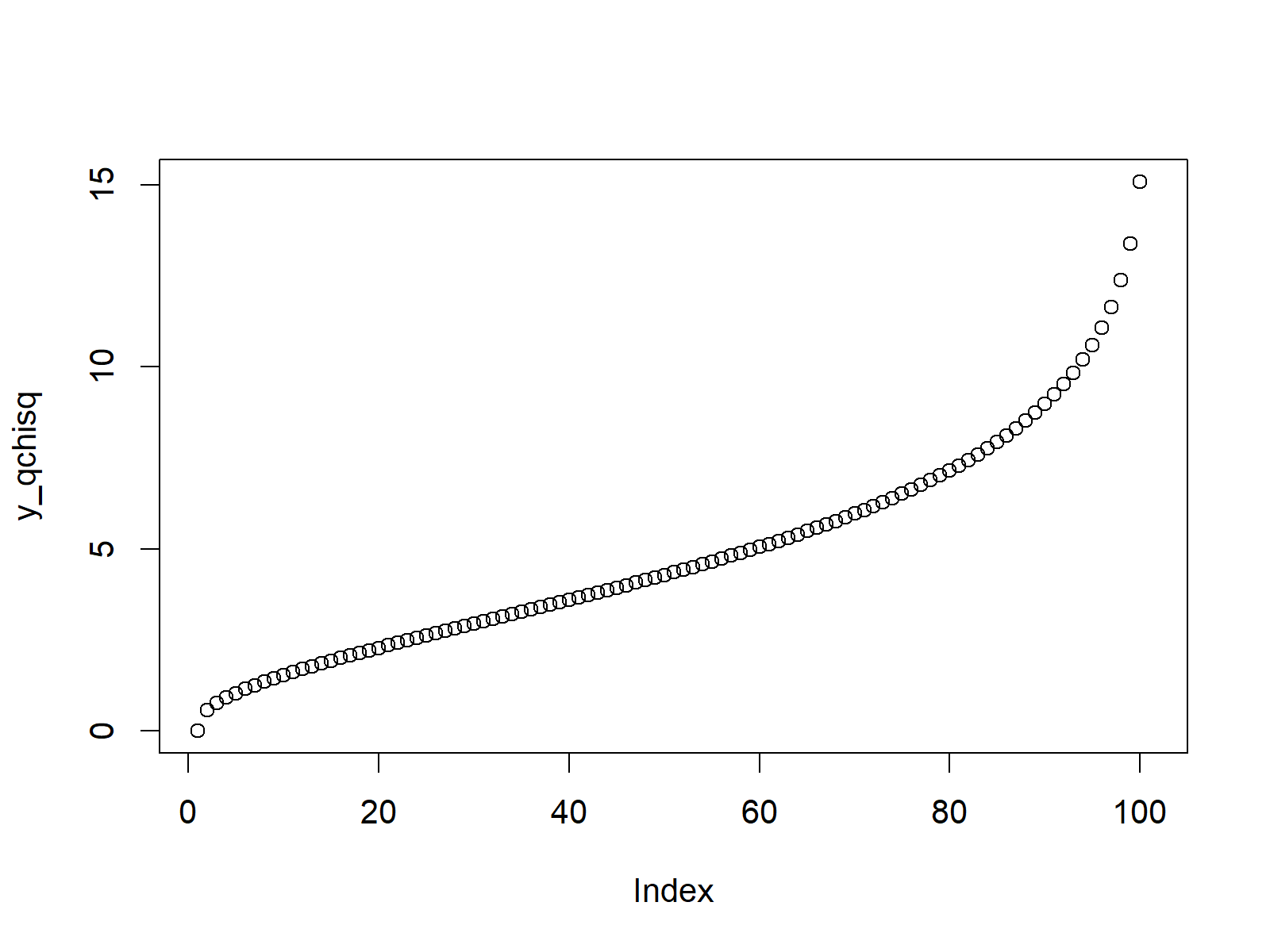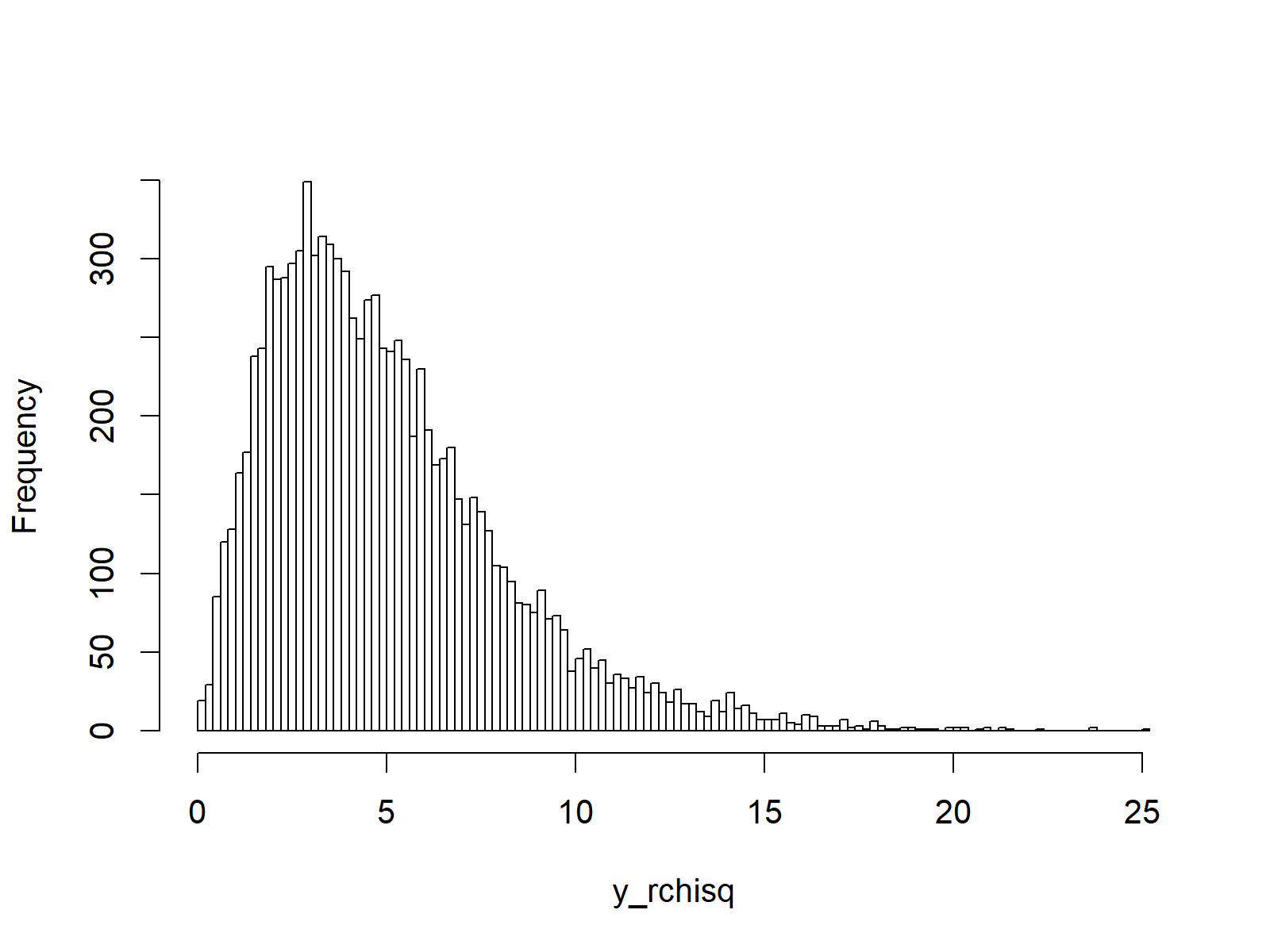# Chi Square Distribution in R (4 Examples) | dchisq, pchisq, qchisq & rchisq Functions

In this R tutorial you’ll learn how to apply the chi square functions.

The content of the article is structured as follows:

Let’s dig in.

## Example 1: Chi Square Density in R (dchisq Function)

In the first example of this tutorial, I’ll show you how to create a density plot of the chi square distribution in R. As a first step, we need to create a sequence of input values:

`x_dchisq <- seq(0, 20, by = 0.1)                         # Specify x-values for dchisq function`

Now, we can apply the dchisq R function to our previously created sequence. Note that we specify the degrees of freedom of the chi square distribution to be equal to 5. You could change this value to produce a chi square density with different degrees of freedom.

`y_dchisq <- dchisq(x_dchisq, df = 5)  # Apply dchisq function`

If we want to create a graphic representing our output, we can use the plot function:

`plot(y_dchisq)                                          # Plot dchisq values`Figure 1: Chi Square Density.

Figure 1 illustrates the chi square plot that we have created with the previous code.

## Example 2: Chi Square Cumulative Distribution Function (pchisq Function)

In the second example, you’ll learn how to create a cumulative distribution function plot of the chi square distribution. First, we need to specify a vector of quantiles (as we already did in Example 1):

`x_pchisq <- seq(0, 20, by = 0.1)                         # Specify x-values for pchisq function`

Now, we can use the pchisq function to this vector to produce our desired values:

`y_pchisq <- pchisq(x_pchisq, df = 5)  # Apply pchisq function`

We can use the plot R function to produce the plot of the cumulative distribution function:

`plot(y_pchisq)                                          # Plot pchisq values`Figure 2: Chi Square Cumulative Distribution Function.

## Example 3: Chi Square Quantile Function (qchisq Function)

Example 3 illustrates how to produce a quantile function plot of the chi square distribution. First, we need to specify a sequence of probabilities (i.e. values between 0 and 1):

`x_qchisq <- seq(0, 1, by = 0.01)                        # Specify x-values for qchisq function`

Then, we can use the qchisq command as follows…

`y_qchisq <- qchisq(x_qchisq, df = 5)  # Apply qchisq function`

…and create our quantile function plot of the chi square distribution as follows:

`plot(y_qchisq)                                          # Plot qchisq values`Figure 3: Chi Square Quantile Function.

## Example 4: Simulation of Random Numbers (rchisq Function)

We can also generate a set of random numbers that are following a chi square distribution. First, we need to specify a seed and a sample size of random numbers that we want to simulate:

```set.seed(53535)                                          # Set seed for reproducibility
N <- 10000                                               # Specify sample size```

Then, we can apply the rchisq function as shown below:

```y_rchisq <- rchisq(N, df = 5)          # Draw N chi squared distributed values
y_rchisq                                                # Print values to RStudio console
# 3.2777584  5.9523747  4.8492891  3.9946643  2.7219484  5.6495423  1.4159975  8.7470479  5.5077211...```

You can see based on the previous RStudio console output how the output vector looks like. Furthermore, we can create a histogram which illustrates the distribution of our random numbers:

```hist(y_rchisq,                                          # Plot of randomly drawn chisq density
breaks = 100,
main = "")```Figure 4: Chi Square Random Numbers.

As you can see based on Figure 4, our random numbers are distributed according to the chi square distribution.

## Video & Further Resources

Do you need more information on the topics of this tutorial? Then you might have a look at the following video of my YouTube channel. In the video, I explain the R syntax of this article:

You might also have a look at the other pages on distributions and the simulation of random numbers in R programming:

Furthermore, you may have a look at the other articles on this website. A selection of related articles is listed below:

Summary: At this point you should have learned how to create and simulate a chi square distribution in R programming. In case you have any further questions, let me know in the comments section.

Subscribe to the Statistics Globe Newsletter